# Learning Cypher with San Francisco Bay Map

## Objectives

The purpose of this exercise to learn graph database and some social network concepts by using commonly known domain: roads and cities. There is no specific problem I need to solve.

## Scenarios and Use Cases

Many of us use Google’s map services everyday to find out what is the best way to go from point-A to point-B, is there an alternative, which way is the fastest or the cheapest. Inspirited by the original graph theory problem: "The paper written by Leonhard Euler on the Seven Bridges of Königsberg and published in 1736 is regarded as the first paper in the history of graph theory", I will use a simplified San Francisco Bay road map to answer some questions. With 3 bridges in my graph, I may be able to ask more interesting questions in future too.I selected some major towns and highways connect them. For simplicity, initially I will only enter part of the data to start the learning.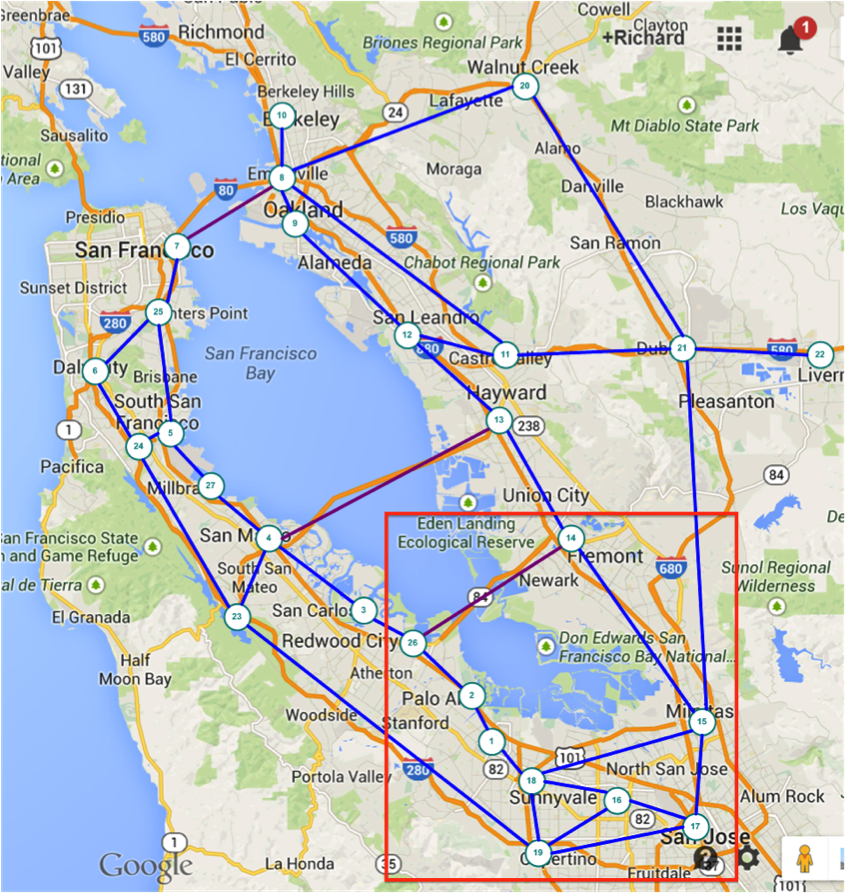## Model

I started with a simple model, just included nodes (cities) and links (highways).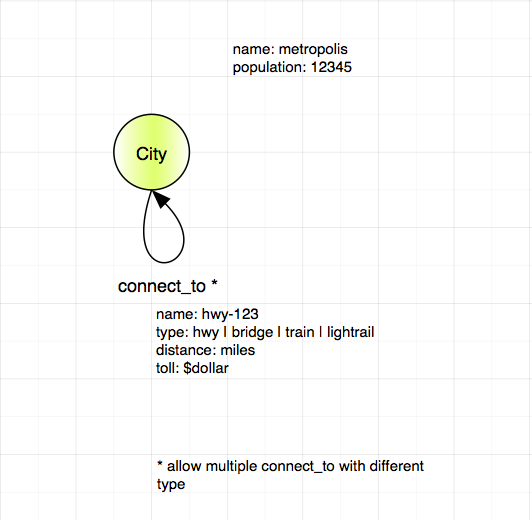Then I added more places, like university, sport stadium and airpor with a relationship [:has] to the city.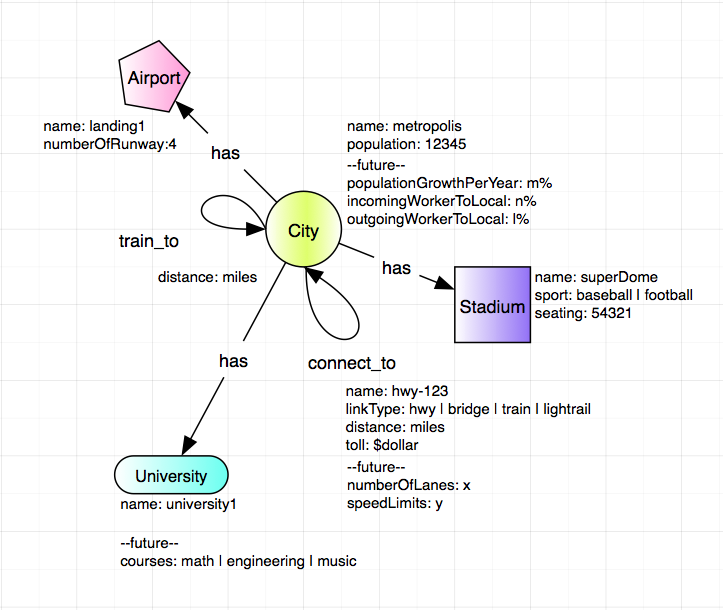``````CREATE (n01:City {name: 'Mountain View', population: 77646}),
(n02:City {name: 'Palo Alto', population: 66642}),
(n18:City {name: 'Sunnyvale', population: 147559}),
(n14:City {name: 'Fremont', population: 224922}),
(n15:City {name: 'Milpitas', population: 69783}),
(n16:City {name: 'Santa Clara', population: 120245}),
(n17:City {name: 'San Jose', population: 998537}),
(n19:City {name: 'Cupertino', population: 60189}),
(n26:City {name: 'Athetron', population: 7159}),

(n01)-[:connect_to {distance: 5.5}]->(n02),		// mtv - pa
(n01)-[:connect_to {distance: 2.9}]->(n18),		// mtv - snyl
(n02)-[:connect_to {distance: 4.2}]->(n26),		// pa - atht
(n26)-[:connect_to {distance: 17.1, linkType: 'bridge', toll: 0}]->(n14),	// atht - frmt
(n14)-[:connect_to {distance: 11.2}]->(n15),		// frmt - mlpt
(n16)-[:connect_to {distance: 5.1}]->(n18),		// stcl - snyl
(n16)-[:connect_to {distance: 4.1}]->(n17),		// stcl - sjs
(n16)-[:connect_to {distance: 7.4}]->(n19),		// stcl - cptn
(n17)-[:connect_to {distance: 11.8}]->(n15),		// sjs - mlpt
(n17)-[:connect_to {distance: 10.3}]->(n19),		// stcl - cptn
(n18)-[:connect_to {distance: 9.9}]->(n15),		// snyl - mlpt
(n18)-[:connect_to {distance: 3.3}]->(n19),		// snyl - cptn
(n02)-[:connect_to {distance: 5.5}]->(n01),		// <<==start reverse direction
(n18)-[:connect_to {distance: 2.9}]->(n01),
(n26)-[:connect_to {distance: 4.2}]->(n02),
(n14)-[:connect_to {distance: 17.1, linkType: 'bridge', toll: 5}]->(n26),
(n15)-[:connect_to {distance: 11.2}]->(n14),
(n18)-[:connect_to {distance: 5.1}]->(n16),
(n17)-[:connect_to {distance: 4.1}]->(n16),
(n19)-[:connect_to {distance: 7.4}]->(n16),
(n17)-[:connect_to {distance: 11.8}]->(n15),
(n19)-[:connect_to {distance: 10.3}]->(n17),
(n15)-[:connect_to {distance: 9.9}]->(n18),
(n19)-[:connect_to {distance: 3.3}]->(n18),
(n17)-[:train_to {distance: 4.0}]->(n16),		// <<==start train
(n16)-[:train_to {distance: 5.1}]->(n18),
(n18)-[:train_to {distance: 2.9}]->(n01),
(n01)-[:train_to {distance: 5.5}]->(n02),
(n02)-[:train_to {distance: 4.2}]->(n26),
(n16)-[:train_to {distance: 4.0}]->(n17),		// dropped 0.1 mile
(n18)-[:train_to {distance: 5.1}]->(n16),
(n01)-[:train_to {distance: 2.9}]->(n18),
(n02)-[:train_to {distance: 5.5}]->(n01),
(n26)-[:train_to {distance: 4.2}]->(n02),
(s01:School {name: 'Stanford University'}),		// <<== add more places
(s02:School {name: 'Foothill Community College'}),
(s03:School {name: 'San Jose State University'}),
(s04:School {name: 'De Anza College'}),
(s05:School {name: 'Santa Clara University'}),
(a01:Airport {name: 'Mineta San Jose International Airport'}),
(n02)-[:has]->(s01), 					// <<== connect places to cities
(n01)-[:has]->(s02),
(n17)-[:has]->(s03),
(n19)-[:has]->(s04),
(n16)-[:has]->(s05),
(n17)-[:has]->(a01)``````

### find out all cities have school

``````// find out all cities have school
MATCH (n:City)-[:has]->(m:School) RETURN n.name, m.name``````

### find out what kind places San Jose has

``````match (n {name: 'San Jose'})-[r:has]->(m)
return n.name, m.name``````

### find the shortest distance from Palo Alto to Santa Clara

``````MATCH p = allShortestPaths((s {name: 'Palo Alto'})-[r:connect_to*..5]->(d {name: 'Milpitas'}))
RETURN NODES(p)``````

### find the shortest route from City A to City B

``````MATCH p=(a {name: 'Palo Alto'})-[r*2..5]->(b {name: 'Milpitas'})
WHERE NOT(a-->b) 			// where a is not directly connected to b
WITH p, relationships(p) AS rcoll 	// just for readability, alias rcoll
RETURN p, reduce(totalDistance=0, x IN rcoll| totalDistance + x.distance) AS totalDistance
ORDER BY totalDistance
LIMIT 1``````

## What I learned?

• This is fun.

• I should use East PaloAlto as a node, instead of Athertron (node-26).

• What need to do next:

• look into the shortestPath algorithm and more complicate queries.

• learn to write API and a web UI to interface server.

## Miscellaneous

The following information may not belong here, but I need to keep them all in one place for now. Since I want to play the graph database in Gephi, so I need to export the data out. GraphML is the file format common to both Neo4j and Gephi. I followed Lorenzo Speranzoni’s Blog-How to load Neo4Art Graph DB into Gephi installed the tool, exported the data to GraphML file and imported into Gephi. I calculated some social network, eccentracity, closeness,etc, it works.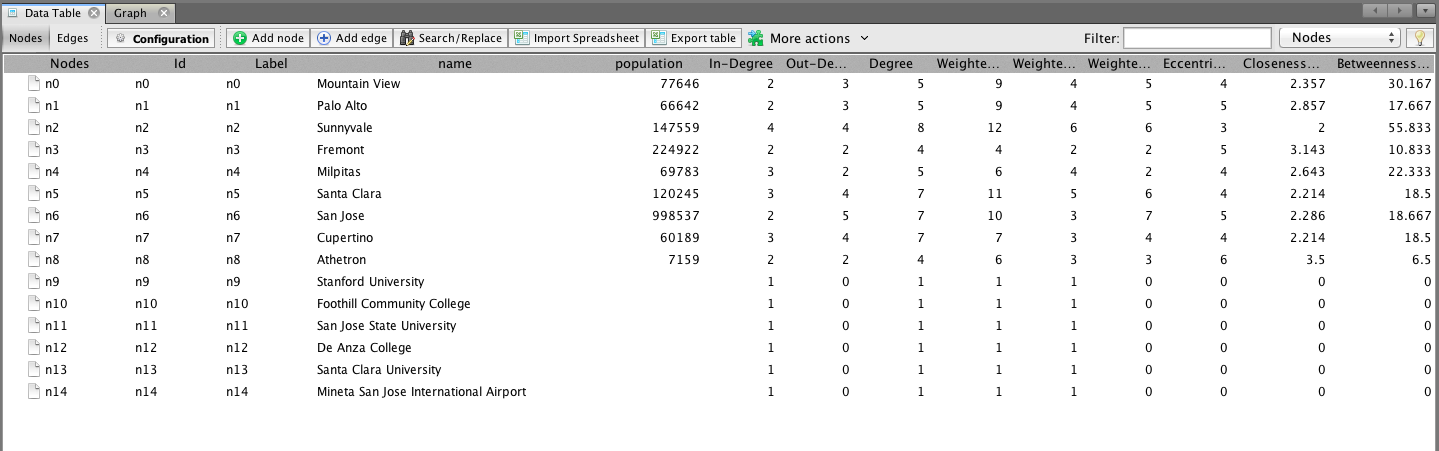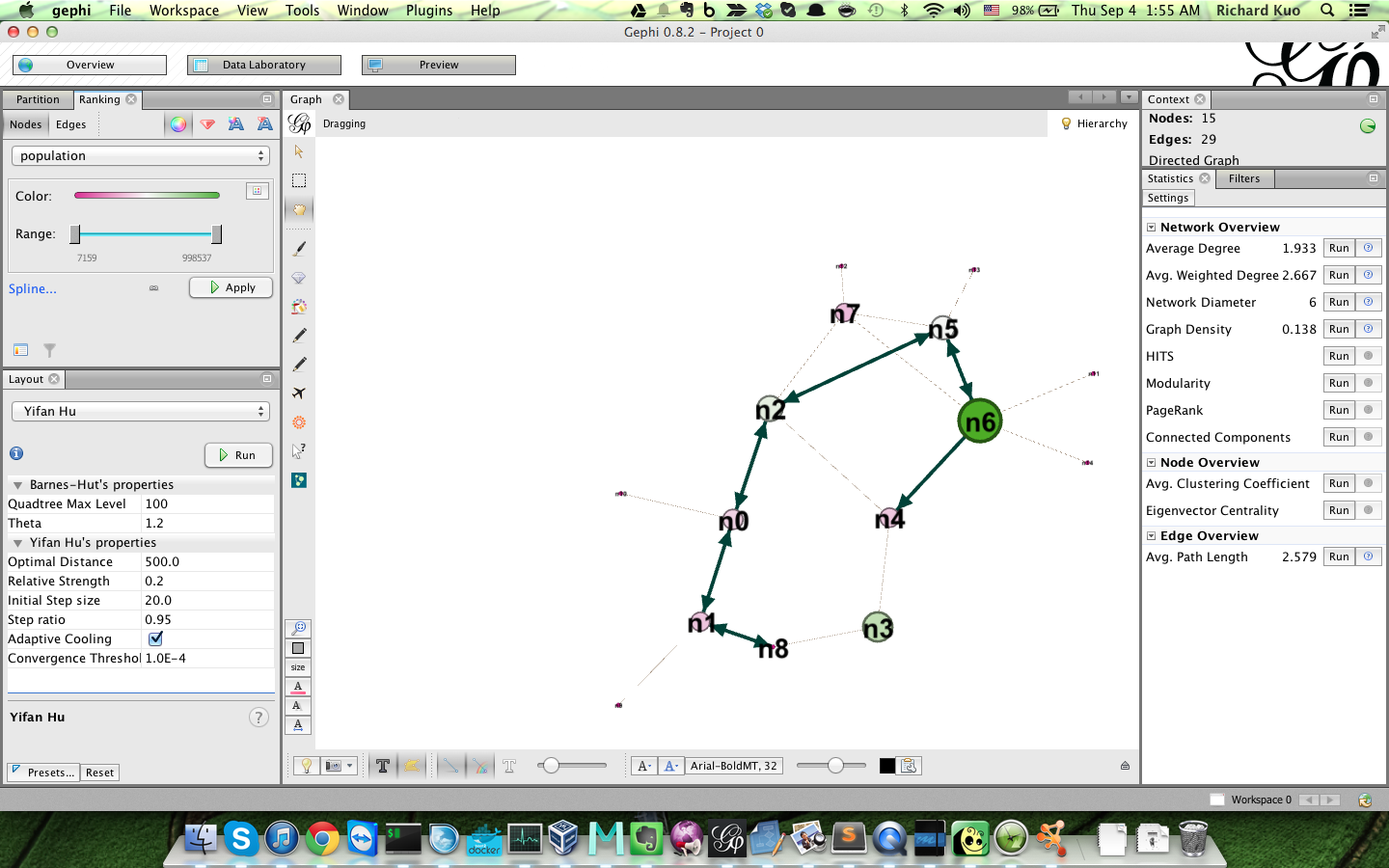I installed Cytoscape and imported the same GraphML file, it works too. Very nice.